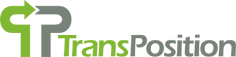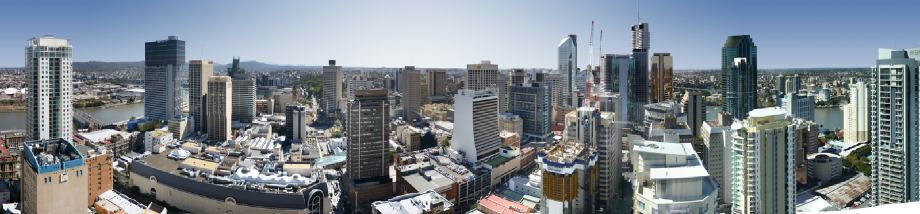# Behavioural Assumptions

The 4S model is based on travellers making decisions that maximise their net utility. The net utility is the utility of their chosen activity at their chosen destination, minus the cost of travelling to that destination. As is usual for utility models, a generalised cost approach is used. A generalised cost approach endeavours to convert all components of travel impedance into dollar cost values. There are three main components of generalised cost; the value of the time spent travelling, the costs of operating the vehicle (including fuel cost, maintenance etc); and any other costs (including fares, tolls, parking etc).

Note that the model uses random distributions for most parameter values. This means that rather than taking a fixed value, the model takes Monte Carlo draws from probability distributions. There are a number of different distributions used by the model, and each distribution is described by a number of key parameters:

• Normal Distribution (mean, standard deviation)
• Log-normal distribution (log-mean, sigma)
• Gamma distribution (mean, sigma or shape, scale)
• Uniform distribution (minimum, maximum)
• Triangular distribution (minimum, midpoint, maximum)

These distributions are given in the text below by showing the name, and the parameters for each distribution.

## Value of Time

The value of time (VOT) attempts to include all factors that influence traveller’s perception of time – including the opportunity costs (foregone wages or the utility of other ways of spending time) and the desirability of spending time on different travel options. This is implemented in the model by determining a basic value of time, and then multiplying time in different travel stages/modes by varying weights. For example, many people would rather spend 10 minutes driving a car than spend 10 minutes walking so, in general, walking is given a higher cost weight than driving. Because it is the dominant mode, driving is given a weight of 1, and the basic value of time is thus the value of time spent driving. Without extensive preference surveys, the basic value of time has been set based on hourly wage rates. The basic wage rate is then factored for different person/trip types.

The distribution of earnings across the community is commonly modelled using one of three possible distributions – exponential, gamma and log-normal . In this model the log-normal distribution has been used.

The value of time is therefore given by:

Basic Value of Time (car drivers) = LogNomal(median=\$/hr, sigma=constant)

The basic value of time is then adjusted using the weighting factors by mode and network characteristic.

For commercial vehicles, the value of time includes a number of components – the value of the driver’s time, the opportunity cost of the vehicle, and the value of time of the freight itself. The wage of a truck driver is much less variable than that of a car driver – car drivers come from a very wide range of occupations and incomes, whereas almost all trucks are driven by people with the same occupation – truck drivers.

We model class 3 (CVM) and class 4 (CVH) vehicles using a linear distribution of the VOT instead of the log-normal distribution we used for class 1 and class 2. A linear approach was used for a number of reasons; firstly driver wages are generally fixed to a small number of awards and these put constraints on how low or high wages can be. Secondly, the time value of the freight itself is variable, but significantly impacted by the quantity of freight carried. Because the model has no assessment of whether commercial vehicles are empty or fully loaded, we use a linear distribution to reflect the range of loaded conditions between empty and full.

## Other Costs

As well as the costs that apply to links, and intersections, there are other costs that apply at the start or end of the trip. These include allowances for the time to park cars and wash up after cycling, as well as the payment of any parking charges.